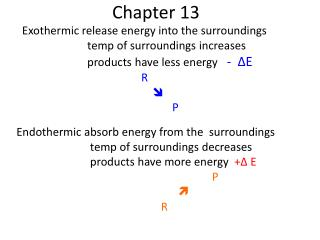# Chapter 13 - PowerPoint PPT PresentationDownload PresentationChapter 13

Chapter 13Download Presentation## Chapter 13

- - - - - - - - - - - - - - - - - - - - - - - - - - - E N D - - - - - - - - - - - - - - - - - - - - - - - - - - -
##### Presentation Transcript

1. Chapter 13 Exothermic release energy into the surroundings temp of surroundings increases products have less energy- ΔE R  P Endothermic absorb energy from the surroundings temp of surroundings decreases products have more energy +ΔE P  R

2. A + B ----> C + D + 30 joules A + B ----> C + D ΔE = -30 joules Forward reaction is exothermic Reverse reaction is endothermic 30 joules + C + D ----> A + B C + D ----> A + B ΔE = + 30 joules

3. Reactions involve • Bond breaking –requires energy +ΔE • Bond formation –releases energy -ΔE • If more energy is needed to break bonds than is released +ΔE ( endothermic) • If more energy is released in bond formation than is absorbed -ΔE (exothermic)

4. Reaction mechanism- steps by which a reaction occurs • Each step of a reaction mechanism involves the collision of two molecules.

5. Colliding molecules need • 1. enough energy to break the bonds • Activation Energy ( A.E) or threshold energy • 2. the proper geometry or orientation (correct angle)

6. Energy (P.E.) reaction coordinate c

7. Factors affecting rate of reaction • Nature of reactants (number of bonds) • Surface area (solids and liquids) • Temperature • Concentration of reactants (solutions and gases) • Catalyst

8. Rate =(# of coll/time) (fract with A.E.) (fract with orientation) • If orientation factor is 1 (orientation does not matter) • TEMPERATURE • affects fraction with A.E.

9. Temp - measure of the average K.E. At a given temp all molecules do not have the same K.E. # of molecules  threshold energy (A.E) Kinetic energy

10. Rate =(# of coll/time) (fract with A.E.) (fract with orientation) • Concentration • affects the # of collisions At a given temp Rate = k (# of collisions /time)

11. Rate = k (# of collisions /time) • k large - fast rxn • k small - slow rxn • Concentration of reactants raised to some power (order) • Rate = (k ) [A]x[B]y • X and Y (orders) • Orders found only by experiment

12. Rate = (k ) [A]x[B]y • If concentration of a reactant is doubled and the rate doubles the order is 1 • If concentration of a reactant is doubled and the rate quadruples the order is 2 • If changing concentration of a reactant has no effect on the rate of reaction it is not included in the rate law • Sum of all orders is the order of the reaction

13. Catalysts and rate • Potential energy Reaction coordinate

14. A catalyst does not change the K.E of the molecules # of molecules • Kinetic energy •  • catalyst no catalyst

15. Catalyst -changes the pathway (steps) Steps require less energy • Changes the orientation requirement- more molecules have required orientation • Not consumed in the reaction

16. Reaction mechanism and Rate Law • Each step involves the collision of two molecules • –adding steps gives net reaction • Each step has its own rate law. • In the steps the coefficients are the orders of the rate law • Slowest step determines the overall rate -rate determining step • The rate law for the slowest step is the rate law for the reaction.

17. Reaction Mechanism • A + B- C + 2D • D+ B  DB • DB + D  F • A + 2B - C + F

18. Determining Reaction Mechanism • 1. Do an experiment to determine the rate law. Experimental rate law • 2. Postulate possible steps by which the reaction could take place. Reaction Mechanism

19. A2+ B2 2AB • rate = k[A2]2 (from experiment) • Possible mechanism 1 • A2+ B2 2AB • rate = k[ A2] [ B2] • Predicted does not match experimental • Not the reaction mechanism

20. A2+ B2 -> 2AB • rate = k[A2]2 (from experiment) • Possible mechanism 2 • Step 1 A 2 + A2  2A + A2 • Step 2 A + B2  AB2 • Step 3 AB2 + A 2AB • Step 1 rate = k[A2][A2] or rate =k[A2]2 • Step 2 rate = k[A][B2] • Step 3 rate = k[AB2][A] • Do any rate laws match the experiment rate law? rate = k[ A2]2

21. 2NO + O2 2NO2